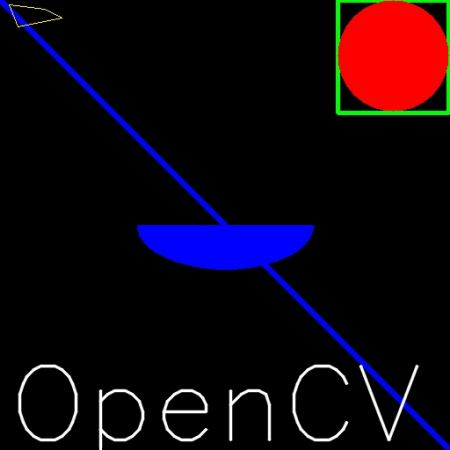OpenCV中的绘图功能¶

目标¶

• 学习使用OpenCV绘制不同的几何形状
• 您将学习以下功能：cv.line()cv.circle()cv.rectangle()cv.ellipse()cv.putText()等。

代码¶

• img：您要绘制形状的图像
• color：形状的颜色。对于BGR，将其作为元组传递，例如：(255,0,0)对于蓝色。对于灰度，只需传递标量值即可。
• 厚度：线或圆等的粗细。如果对闭合图形（如圆）传递-1 ，它将填充形状。默认厚度= 1
• lineType：线的类型，是否为8连接线，抗锯齿线等。默认情况下，为8连接线。**cv.LINE_AA**给出了抗锯齿的线条，看起来非常适合曲线。

画线¶

import numpy as np
import cv2 as cv
# 创建黑色的图像
img = np.zeros((512,512,3), np.uint8)
# 绘制一条厚度为5的蓝色对角线
cv.line(img,(0,0),(511,511),(255,0,0),5)

画椭圆¶

cv.ellipse(img,(256,256),(100,50),0,0,180,255,-1)

画多边形¶

pts = np.array([[10,5],[20,30],[70,20],[50,10]], np.int32)
pts = pts.reshape((-1,1,2))
cv.polylines(img,[pts],True,(0,255,255))

向图像添加文本：¶

font = cv.FONT_HERSHEY_SIMPLEX
cv.putText(img,'OpenCV',(10,500), font, 4,(255,255,255),2,cv.LINE_AA)

结果¶